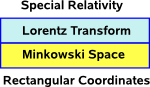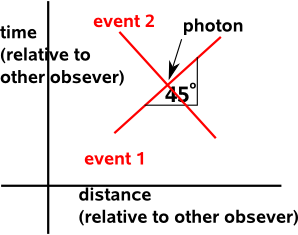# Physics - Speed of Light

If we think of a photon as an electromagnetic wave then we would expect them to be explained by Maxwell's equations, that is the magnetic field is proportional to the rate of change of the electric field and the electric field is proportional to the rate of change of the magnetic field. There is nothing in this to limit the speed of the wave (no equivalent of inertia), also we would expect the wave to spread out, this was a mystery before Einstein.

On this page we will investigate the idea (in a non-rigorous and speculative way) that, to an external observer a photon is a line along an axis that squares to zero and in the frame of the photon it is a point.

Image that an event happens, perhaps an electron changes energy level, and a photon is generated this will then travel through space and time (relative to some observer) until another event destroys it.To illustrate this I have drawn a diagram showing time against distance. We have chosen our distance vector so that the photon is traveling along it.

### Units

I have chosen the units such that c=1, this means that we either measure distance in seconds or time in metres, this is not so strange: astronomers often measure distance in light-years. This system of units has some advantages: E=mc² becomes E=m, the Lorentz transform has one type of unit and photons always travel at 45° on the above diagram. Perhaps I should not use the word 'travel' because time is one of the dimensions so might be better to say that the photon is represented as a line on this diagram.

## Minkowski and Lorentz

There are two sources of 'curvature' in special relativity which we could think of as layers:

• Minkowski Space - adds time as a 4th dimension (time) which squares to a different sign than space which leads to curvature.
• Lorentz Transforms - This adds an additional distortion caused by the relative velocity of the observer.

We can think of these distortions as 'layers' on top of flat space:We will consider these layers separately:

## Minkowski Space

We can consider Minkowski space as a four dimensional space where either, 3 dimensions square to positive and one dimension squares to negative or 3 dimensions square to negative and one dimension squares to positive. So for these proposes lets choose the first:

• space - 3 dimensions which square to positive.
• time - 1 dimension which squares to negative.

We can now look at transforms (translation and rotation) in our plane containing one space and one time dimension. This is worked out on this page which gives the following results:

We do these :

1. Take the exponent of both inputs.
2. Multiply these exponents together.
3. Take the inverse exponent = ln = loge of the result.

The way we distinguish between translations and rotations is that we use double numbers for one and complex numbers for the other:

2D Euclidean space
(2 space dimensions)

2D Minkowski space
(1 space and 1 time)
translations double numbers complex numbers
rotations complex numbers double numbers

Where:

So what is the effect of rotation in our 2 dimensional plane? Rotating the line representing the particle changes its speed:What is the effect of translation in our 2 dimensional plane? This does not effect the points the particle passes through (unless we also transform the events at the end of line) what it does alter is when the particle passes through each point.As we can see from the table above, this translation is done using complex numbers, in other words it has the same properties as rotation in Euclidean space. This seems to imply that a moving particle has wave-like properties.

## Lorentz Transform

The Lorentz transform relates the spacetime coordinates (t,x) to (t',x'), spacetime in frames traveling at different speeds.

Assuming the relative motion 'v' is along the x dimension then we can simplify the Lorentz transform to 1 space and 1 time dimension so the transform (known as a 'boost') will be:

 ct' x'
=
 γ -ßγ -ßγ γ
 ct x

where:

• t,x = distance and time when not moving
• t',x' = distance and time with relative velocity
• γ = 1/√(1 - ß2)
• ß = v/c
• v = relative velocity
• c = speed of light

### In the frame of a Photon

So how would this look in the frame of the photon? When the observer is traveling at light speed. So when the observer is traveling with the light beam then v=c so ß = 1 and γ = 1/sqrt(0) = ∞

So at light speed the Lorentz transform is:

 ct' x'
= ∞
 ct x

So I would interpret this as saying either:

• In the frame of reference of the photon the time and space of other observers is zero, or,
• In the frame of reference of other observers the time and space of the photon is infinite.

I think I prefer the first option, if we use this and ask the question, what is it like to ride on a light beam? Then I think the answer might be that we can't know because, in that frame, the photon is created and destroyed instantaneously. In that frame it connects the endpoints of the line together.

So how can we redraw the above diagram in the frame of reference of the photon? what would the events become? would other photons still be lines?Would perhaps events change from points to lines and photons from lines to points? Here I am into pure speculation and I suspect this is wrong.

### Faster Than Light

This page does not have enough theory to answer questions about how we might be able to get particles to go faster than light or what energy that would take. But we can use the Lorentz transform again to relate the spacetime coordinates (t,x) to (t',x'), spacetime in frames traveling at different speeds assuming this is still valid when v>c.

Lets assume that the observer is traveling at v=√2c so ß = √2 and γ = 1/√(1 - 2) = 1/i =-i

So at light speed the Lorentz transform is:

 ct' x'
=
 -i i√2 i√2 -i
 ct x

## Mathematical Spaces

So how does this relate to the idea that time and space dimensions square to -ve and +ve values (or visa versa)? That is Minkowski space?

We have one dimension that squares to +ve (say a space dimension) and another dimension that squares to -ve (say time). If we rotate then, between these at 45°, we will have dimensions that square to zero.It is tempting to relate this to the diagram at the top of this page and I think we might be able to, but we must be careful, as there are some differences between the diagrams.

The diagram at the top of the points represents the absolute position in space-time and so events can be translated (offset from the origin). However in this diagram the dimensions must go through the origin. I think we need a way to add and remove translations, one way to do this might be to work with difference values, which is all we need for velocities?

Also I'm not completely clear about the mathematics of this, it makes intuitive sense that if the vertical and horizontal square to +ve and -ve then, if we rotate them, somewhere inbetween there will be an axis that squares to zero and this does seem to be an established mathematical concept (lookup 'null vectors').

When I try this with complex numbers I get:

• real axis 'a' squares to a² (right hand side of real axis)
• imaginary axis 'a i' squares to -a² (left hand side of real axis)
• forward diagonal 'a+a i' squares to ia² (upper imaginary axis)
• forward diagonal 'a- a i' squares to -ia² (lower imaginary axis)

So the diagonals don't square to zero, I think that's because real and imaginary parts of complex numbers don't anti-commute, or to put it another way complex numbers consist of real and bivector parts and we require vector parts.

I think if we use:

a e1 + a e2

where: e1²=1 and e2²=-1

then (a e1 + a e2)²=0

## Summary

This page proposes some ideas in a non-rigorous way and I would appreciate any ideas to take this forward.

Where I can, I have put links to Amazon for books that are relevant to the subject, click on the appropriate country flag to get more details of the book or to buy it from them.Deep Down Things: The Breathtaking Beauty of Particle Physics - If you dont want any equations then this is a good and readable introduction to quantum theory and related mathematics such as Lie groups, Gauge Theory, etc.

Commercial Software Shop

Where I can, I have put links to Amazon for commercial software, not directly related to the software project, but related to the subject being discussed, click on the appropriate country flag to get more details of the software or to buy it from them.

This site may have errors. Don't use for critical systems.